# Logical Reasoning - Test 1

## 25 Questions MCQ Test SSC CGL Tier 1 Mock Test Series | Logical Reasoning - Test 1

Description
Attempt Logical Reasoning - Test 1 | 25 questions in 15 minutes | Mock test for SSC preparation | Free important questions MCQ to study SSC CGL Tier 1 Mock Test Series for SSC Exam | Download free PDF with solutions
QUESTION: 1

### Select the related word from the given alternatives. BIRD : AEROPLANE : : FISH : ?

Solution:

A bird’s mode of transport is through the air. The man-made creation that serves a similar purpose is the aeroplane.
Similarly, a fish’s medium of transport is through water. The corresponding man-made mode of transport is a boat.

QUESTION: 2

### Select the related letters from the given alternatives. DCBA : WXYZ : : IJKL : ?

Solution:

The first phrase consists of letters written in reverse alphabetical order (D → C → B → A). The corresponding phrase is in regular alphabetical order (W → X → Y → Z).
Similarly, the given phrase is in regular alphabetical order (I → J → K → L). Therefore, the required word must be in reverse alphabetical order.
Further, the last letter of the first phrase (A) is the first letter of the alphabet, and the last of the second word (Z) is the first in reverse alphabetical order.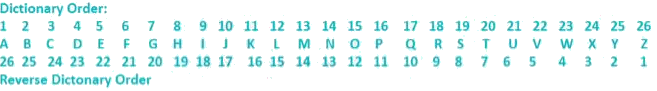L is the 12th in regular order, and therefore the last letter of the required word must end with the same position in reverse order, i.e. O.
Therefore, the required word is RQPO.

QUESTION: 3

### Select the related numbers from the given alternatives. 381 : 160 : : 478 : ?

Solution:

The difference between the first pair is 221, i.e. 381 – 160 = 221.
Therefore, the required answer will be 478 – 221 = 257.

QUESTION: 4

Find the odd word from the given alternatives.

Solution:

Affluent, Rich and Well-to-do all refer to high level of material wealth. However, prestigious refers more to stature or achievements than material wealth.

QUESTION: 5

Find the odd letters from the given alternatives.

Solution:

By rearranging the letters such that the second and first place are interchanged, the letters should be every alternate letter in the alphabet.
1) OMQ → MOQ → M N O P Q
2) TPR → PTR → P Q R S T → Does not satisfy.
3) TRV → RTV → R S T U V
4) VTX → TVX → T U V W X
Therefore, TPR is the odd answer.

QUESTION: 6

Find the odd number pair from the given alternatives.

Solution:

There is a difference of three between each pair.
11 – 8 = 3, 4 – 1 = 3, 10 – 7 = 3.
However, 5 – 3 = 2, and therefore this is the odd one out

QUESTION: 7

Arrange the following words as per order in the dictionary.
1. Launch, 2. Laugh, 3. Lattice, 4. Latent, 5. Latitude

Solution:

Words in dictionary order are listed such that they are in alphabetical order on the basis of the first letter they differ in. Therefore, the order will be :
Latent (4), Latitude (5), Lattice (3), Laugh (2), Launch (1).

QUESTION: 8

A series is given, with one term missing. Choose the correct alternative from the given ones that will complete the series.

XFB, UGD, RIG, OLK, ?

Solution:

The relation between the letters is as follows,
X – 3 = U, U – 3 = R, R – 3 = O, O – 3 = L
F + 1 = G, G + 2 = I, I + 3 = L, L + 4 = P
B + 2 = D, D + 3 = G, G + 4 = K, K + 5 = P
Hence the next term is LPP.

QUESTION: 9

A series is given, with one term missing. Choose the correct alternative from the given ones that will complete the series.

1, 2, 4, 7, ?, 16

Solution:

The difference between each increases by one.
2 – 1 = 1
4 – 2 = 2
7 – 4 = 3
Therefore, the required number will be 7 + 4 = 11, or 16 – 5 = 11.

QUESTION: 10

A & B are married couple. X and Y are brothers. A is the brother of X. How is Y related to B?

Solution: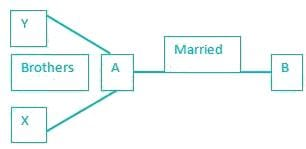therefore, since X and Y are brothers, and X and A are brothers, Y and A are brothers. This makes Y the brother in law of B.

QUESTION: 11

A is shorter than B but taller than C. D is shorter than A but taller than C and E is shorter than B but taller than A. The shortest person is ?

Solution:

1) A is shorter than B but taller than C.
⇒ C < A < B
2) D is shorter than A but taller than C.
⇒ C < D < A < B
3) E is shorter than B but taller than A.
⇒ C < D < A < E < B
Therefore, C is the shortest.

QUESTION: 12

From the given words, select the word which cannot be formed using the letters of the given word.

DISSERTATION

Solution:

Let us consider each option.
1) DISSERTATION → SISTER
2) DISSERTATION → STATION
3) DISSERTATION → DIRECTION
4) DISSERTATION → RATION.
There is no ‘C’ in dissertation, and therefore, direction cannot be formed.

QUESTION: 13

If A = 1, E = 5, then HEAR = ?

Solution:

Each letter is denoted by its corresponding numeric place in the alphabet.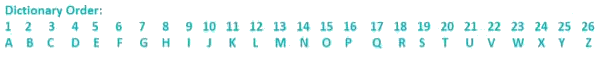Therefore, H = 8, E = 5, A = 1 and R = 18.
On adding these, we get 8 + 5 + 1 + 18 = 32.

QUESTION: 14

If P denotes +, Q denotes - , R denotes '÷', and S denotes ×, then : 18S36R12Q6P7 = ?

Solution:

By putting in the required operators into the system, we get :
18 × 36 / 12 – 6 + 7
On applying BODMAS, we get
18 x (36/12) – 6 + 7
= (18 x 3) – 6 + 7
= 54 + 1
= 55

QUESTION: 15

Some equations have been solved on the basis of certain system. Find the answer for the unsolved equation on that basis. If

98 - 39 - 27 = 31,
87 - 38 - 34 = 20,
then 79 - 25 - 12 = ?

Solution:

Here the logic is as follows,
9 × 8 = 72, 3 × 9 = 27, 2 × 7 = 14
⇒ 72 – 27 – 14 = 31
8 × 7 = 56, 3 × 8 = 24, 3 × 4 = 12
⇒ 56 – 24 – 12 = 20
Similarly,
7 × 9 = 63, 2 × 5 = 10, 1 × 2 = 2
⇒ 63 – 10 – 2 = 51
Hence the required number is 51.

QUESTION: 16

Select the missing number from the given responses :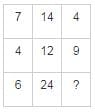Solution:

Here the relation is as follows : column 1 × √column 3 = column 2
Thus,
7 × √4 = 7 × 2 = 14
4 × √9 = 4 × 3 = 12
Similarly,
6 × √x = 24
√x = 24/6
x = 42
x = 16
Hence the required number is 16.

QUESTION: 17

Rahul walks 30 meters towards south. Then turns to his right and starts walking straight till he completes another 30 meters. Then again turning to his left he walks for 20 meters. He then turns to his left and walks for 30 meters. How far is he from his initial position?

Solution: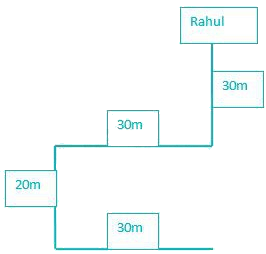Since Rahul has walked 30 meters to the right, and later 30 meters to the left, he is back in the same line which he originally started in.
Therefore, the total distance from the initial point will be given by the distance he covered towards the south, i.e. 30 + 20 = 50 meters.

QUESTION: 18

You have to consider the statement to be true, even if it seems to be at variance from commonly known facts. You have to decide which of the given conclusions, if any, follow from the given statements.
Statements:
All teachers are actors.
Some actors are women.
Conclusion:
I. All teachers are women.
II. Some women are teachers.
III. Some women are actors.
IV. All actors are teachers.

Solution:

each statement after analyzing the least possible Venn diagram.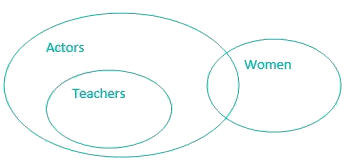Conclusions:
I. All teachers are women → It’s possible but not definite, hence false.
II. Some women are teachers → It’s possible but not definite, hence false.
III. Some women are actors → It’s definite, hence true.
IV. All actors are teachers → It’s possible but not definite, hence false.
Therefore, only conclusion III will follow.

QUESTION: 19

How many triangles are there in the following figure PQRS?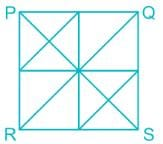Solution:

Let us check the number of triangles formed,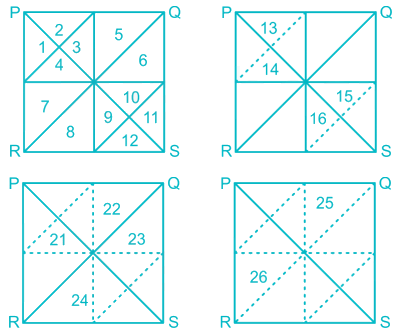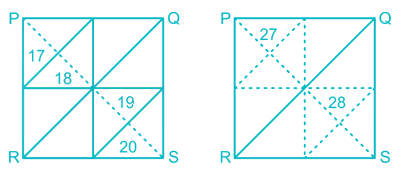Hence 28 triangles are formed.

QUESTION: 20

Identify the diagram that best represents the relationship among classes given below : Stationery items, Pencils, Potatoes

Solution:

As we all know Pencil comes under Stationery items whereas Potatoes is an edible item.

So the correct Venn diagram is as follows,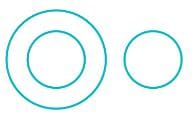QUESTION: 21

Which answer figure will complete the pattern in the question figure?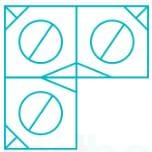Solution:

The required image should have circle in middle with a diameter from north - east to south - west. There should be a slanting line at upper left corner and a ‘T’ at lower right corner.

Hence the required image is as shown,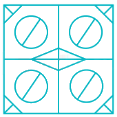QUESTION: 22

From the given answer figures, select the one in which the question figure is hidden/embedded.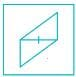Solution:

The image given in question is hidden in the following image as shown,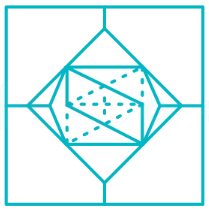QUESTION: 23

A piece of paper is folded and cut as shown below in the question figures. From the given answer figures, indicate how it will appear when opened.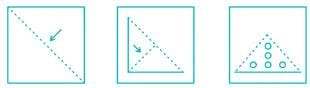Solution:

When we open the paper step by step, we get the following figure.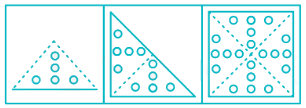QUESTION: 24

If a mirror is placed on the line MN, then which of the answer figures is the right image of the given figure?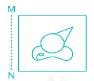Solution:

The mirror image of the given figure is as shown,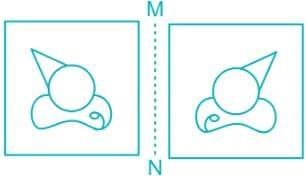QUESTION: 25

In this question, the sets of numbers given in the alternatives are represented. The columns and rows of Matrix I are numbered from 0 to 4 and that of Matrix II are numbered from 5 to 9. A letter from these matrices can be represented first by its row and next by its column, e.g., A can be represented by 02, 14, etc., and 'O' can be represented by 55, 67, etc. Similarly you have to identify the set for the word 'LEND'.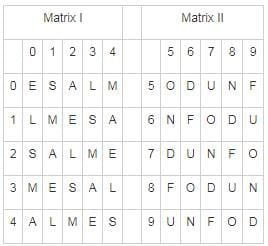Solution:

Let’s check each option,
1) 34, 43, 40, 56 → LEAD
2) 10, 31, 77, 99 → LEND
3) 41, 12, 41, 59 → LELF
4) 22, 14, 65, 75 → LAND
Hence the correct representation of the word LEND is 10, 31, 77, 99.Use Code STAYHOME200 and get INR 200 additional OFF Use Coupon Code